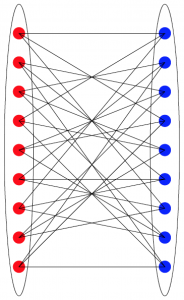Descriptions of the three research groups are given below.

Statistics:  An Investigation of the Realistic Application of the Kelly Criterion
Professor Trent Gaugler

In any scenario in which a person invests money, whether it be investing in the stock market or in the outcome of a sporting event, there is obvious interest in developing a strategy that will maximize long-term returns.  Many pundits argue over the validity of certain betting systems, but much of this is based on speculation and anecdotal evidence. The theoretical properties of betting systems, including progressive betting systems like the Kelly Criterion, have been studied, but in reality many of the assumptions on which such analyses rely are untenable.  Moreover, many theoretical analyses are done in a manner that does not mimic real-world investing.  For example, many investigations into progressive betting systems use sequences of events that occur one at a time, while in reality several events will be ongoing at once – that is, you may invest in several companies simultaneously, or you may wager on the outcomes of several games that are played concurrently.  A principled investigation of the realistic application of such a betting system is needed to understand its practical properties.

Our group will undertake an intensive empirical investigation by focusing on the use of the Kelly Criterion for betting on college football games.  In order to investigate the Kelly Criterion in this setting, we must first tackle the task of finding good estimators of the parameters in the Kelly formula, namely the probabilities of outcomes of games.  The development of a model to predict outcomes of games will necessitate some data retrieval and cleaning, and the predictions will tune the betting process.  We will also study variations on the Kelly betting system to understand the relationship between total returns and volatility.

Applicants must have some facility with computer programming, as demonstrated by having gained proficiency with a language like R, Python, C, or similar; familiarity with R will be viewed favorably.  Applicants must also have basic knowledge of probability and statistics, while knowledge of linear models and logistic regression would be helpful.

Combinatorics and Algebra:  Algebraically Defined Graphs and Incidence Geometry
Professor Brian Kronenthal

This research group will study properties of certain bipartite graphs in which each vertex is given an $$(x,y)$$-coordinate pair, and two vertices are adjacent when their coordinate pairs satisfy an equation.  For example, one could study a graph in which $$(x_1,y_1)$$ is adjacent to $$(x_2,y_2)$$ whenever $$x_2 + y_2 = x_1^2 y_1^5 + x_1 y_1^3$$.

In particular, we will be interested in the properties of cycles that appear in these graphs.  This is equivalent to studying when special equations, or systems of special equations, have solutions.  We will explore how these properties change when the coordinates are subject to different restrictions, as well as when we generalize the graphs in various ways.

Questions related to this project have applications to incidence geometry, and in particular to structures called projective planes and generalized quadrangles. However, prior knowledge of incidence geometry, projective planes, and generalized quadrangles is neither assumed nor required.

Applicants should have taken a course in abstract algebra; a course in number theory may be substituted in exceptional cases.  A course in combinatorics (or graph theory) and programming experience may prove valuable, but are not required.  Most importantly, students should have tenacity, creativity, and be interested in working with systems of equations and bipartite graphs.Geometry and Number Theory:  The Geometry of Gaussian Primes
Professor John Meier

While there are many prime numbers that are close together (11 and 13, 17 and 19, and so on), there are also stretches of integers that contain no primes at all. Because of these gaps, there is no way to “walk to infinity” by stepping only on prime numbers.  To make this a bit more precise, consider the case where you can stride up to 5 units.  Then you could step $2 \rightarrow 3 \rightarrow 5 \rightarrow 7 \rightarrow 11 \rightarrow 13 \rightarrow 17 \rightarrow 19 \rightarrow 23$ but you cannot progress any further, because the next prime is 29, which is 6 units away.  There are similar gaps for strides up to 6, or 7, or in fact $$n$$ units. Because for any integer $$n$$ there are no primes in the sequence $(n+1)! + 2, \, (n+1)! + 3, \, … , \, (n+1)! + (n+1)$

The Gaussian integers are complex numbers $$a + b\textbf{i}$$, where $$a$$ and $$b$$ are integers, hence the Gaussian integers correspond to the integer lattice points in the complex plane.  The subset of Gaussian primes is then a collection of points in the integer lattice, and as such, one can ask any number of questions about the geometry of this subset.  There are a few very interesting results about the distribution of Gaussian primes in the literature.  For example, it is known that there are large moats in the distribution of Gaussian primes.  That is, there are large circular regions in the complex plane which contain no Gaussian primes.  On the positive side, it is also known that many constellations must exist among the Gaussian primes: given any set of Gaussian integers $$\{i_1, i_2, \ldots, i_k\}$$ there is a Gaussian integer $$a$$ and an ordinary integer $$m$$ such that $$\{a+ i_1 m, a + i_2 m, \ldots, a+ i_k m\}$$ is contained in the Gaussian primes.  In fact, there are infinitely many such values of $$a$$ and $$m$$ that will transform the original set of Gaussian integers into Gaussian primes.

Guided by prior results like those mentioned above, our group will explore the geometry of Gaussian primes using a mixture of theoretical mathematics along with empirical investigation.

Applicants must have completed a course in linear algebra.  Prior knowledge of topics in number theory and geometry may be helpful, but is not necessary.  Facility with computer algebra systems (like Mathematica) will be helpful, but is also not necessary.